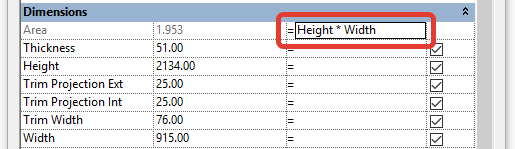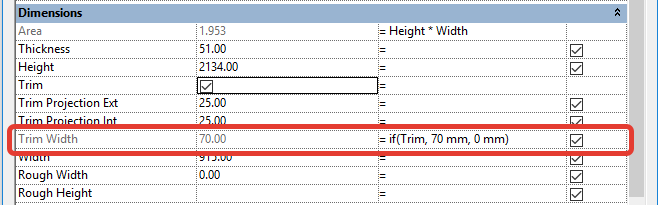# Revit Formulas and Conditionals – Getting to know Revit Formulas!

Revit Formulas are powerful ways to drive and control parametric content in a model. If you are building Revit Families, you have probably come across the need to add formulas. These Revit formulas range from the simple to very complex! If you’re looking for info on what Formulas are available to you and how to use them, you have come to the right place. My friend Chris Alby from measuresurvey365.co.uk  is here to explain all about Revit Formulas!## Formula syntax

Revit formulas support standard arithmetic and trigonometric functions.

The following operators and functions are used in formulas:

• Subtraction: –
• Multiplication: *
• Division: /
• Exponentiation: ^ (Example: 3 ^ 2 is 3 squared)

All of the following functions have a standard notation: function (value)

For example: sin (Angle) is the sine of the Angle parameter.

• Logarithm: log
• Square root extraction: sqrt
• Sine: sin
• Cosine: cos
• Tangent: tan
• Arcsine: asin
• Arccosine: acos
• Arctangent: atan
• 10 to the power of x: exp (x)
• Number modulus: abs
• Pi number: pi ()

Parameter names in formulas are case sensitive. If the parameter name begins with a capital letter, for example “Width”, then it should be entered in the formulas starting with a capital letter.

Examples of using functions in formulas:

• Length = Height + Width + sqrt (Height * Width)
• Length = Length1 + Length2
• Area = Length * Width
• Area of ​​a Circle = pi () * Radius ^ 2
• Volume = Length * Width * Height
• Width = 100 mm * cos (Angle)
• Parameter = 2 * abs (a) + abs (b / 2)
• Number of array elements = Length / StepIf you need to block the value of any parameter from changing from the project, its value is written in the formula (for text, the value is written in quotes):You can use the round function for values ​​in formulas.

round (x) The round function returns the value rounded to the nearest integer. This does not take into account the direction of rounding. round (3.1) = 3, round (3.5) = 4

roundup (x) The roundup function returns a value rounded to the maximum integer value greater than or equal to x. roundup (3) = 3, roundup (3.1) = 4

rounddown (x) The rounddown function returns the value rounded to the minimum intrinsic value less than or equal to x. rounddown (3) = 3, rounddown (3.7) = 3

Rounding only works with numbers without dimensions, i.e. does not work with parameters of length, area, etc.

## Conditional Expression Syntax

You can use conditional expressions in Revit. Conditional expression structure:

IF (<condition>, <value if satisfied>, <value if not satisfied>)

Example: if (Area> 1 m2, 500 mm, 300 mm)When using parameters of the “Yes / No” type, the form of the condition is shortened:The following comparison operators can be used in a condition: “<“, “>”, and “=”. Boolean operators are also supported: “AND”, “OR” and “NOT”.

If you need to parameterize a parameter of the “Yes / No” type, a shortened notation is also used.Revit Formulas are well worth knowing about, especially when you get into Parametric Family Creation. Do you have any great tips about Revit Formulas? Please share in the comments below!

#### Article written by: Chris Alby

Chris Alby is an Architect who has been working in Revit for over 8 years. He blogs about Revit at: measuredsurvey365.co.uk And he also shares free Revit families